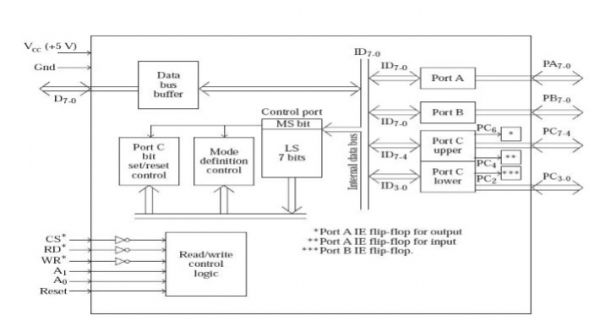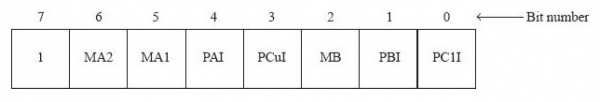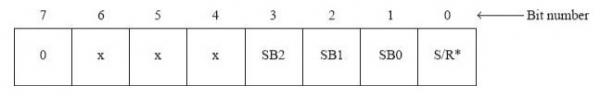# Control port of 8255

In 8255, there are two types of command words or control word are available. And they are −

• Mode definition control word and

• Port C bit set/reset control word.

Both these are written to the control port only. From the point of view of the microprocessor there is a single 8-bit control port, which is selected when CS* = 0, WR* = 0, A1 = 1 and A0 = 1. But if we consider the architecture of 8255, internally there are two control ports, one for mode definition control and another for Port C bit set/reset control. The contents of the control port get latched in mode definition control port if the MS bit of control port = 1. If the MS bit of control port = 0, the contents of the control port gets latched in Port C bit set/reset control port. This can be seen from the following fig.Now let us discuss about the Mode Definition Control Word. The following fig depicts the same −The following table depicts the meaning and purposes for all these bits −

 Bit 0 (PClI) 1 = Port C lower (PC3-0) as input0 = Port C lower (PC3-0) as output Bit 1 (PBI) 1 Port B as input0 = Port B as output Bit 2 (MB) 1 = Port B in mode 10 = Port B in mode 0 Bit 3 (PCuI) 1 = Port C upper (PC7-4) as input0 = Port C upper (PC7-4) as output Bit 4 (PAI) 1 = Port A as input0 = Port A as output Bits 6, 5 0 0 = Port A in mode 0 (MA2,MA1) 0 1 = Port A in mode 11 0 = Port A in mode 21 1 = Port A in mode 2 Bit 7 Must be 1 to indicate that it is mode definition control.

Now let us discuss about the Port C Bit Set/Reset C4 Word. The following fig depicts the same −The following table depicts the meaning and purposes for all these bits −

 Bit 0 (S/R*) 1 = Set Port C bit selected by bits 3, 2, and 10 = Reset Port C bit selected by bits 3, 2, and 1 Bits 3, 2, 1 (SB2, 1, 0) 000 = Select bit 0 of Port C001 = Select bit 1 of Port C010 = Select bit 2 of Port C011 = Select bit 3 of Port C100 = Select bit 4 of Port C101 = Select bit 5 of Port C110 = Select bit 6 of Port C111 = Select bit 7 of Port C Bits 6, 5, 4 Are don't cares. Generally loaded with 000 Bit 7 0 to indicate it is Port C bit set/reset control